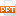# Bài giảng Functions and models - 1.3 New Functions from Old Functions

This means we obtain the desired graph by starting with the parabola y = x2 and shifting 3 units to the left and then 1 unit upward.24 trang | Chia sẻ: vutrong32 | Ngày: 20/10/2018 | Lượt xem: 325 | Lượt tải: 0
Bạn đang xem trước 20 trang tài liệu Bài giảng Functions and models - 1.3 New Functions from Old Functions, để xem tài liệu hoàn chỉnh bạn click vào nút DOWNLOAD ở trên
1.3New Functions from Old FunctionsIn this section, we will learn:How to obtain new functions from old functions and how to combine pairs of functions.FUNCTIONS AND MODELSIn this section, we:Start with the basic functions we discussed in Section 1.2 and obtain new functions by shifting, stretching, and reflecting their graphs. Show how to combine pairs of functions by the standard arithmetic operations and by composition.NEW FUNCTIONS FROM OLD FUNCTIONSBy applying certain transformations to the graph of a given function, we can obtain the graphs of certain related functions.This will give us the ability to sketch the graphs of many functions quickly by hand.It will also enable us to write equations for given graphs.TRANSFORMATIONS OF FUNCTIONSLet’s first consider translations.If c is a positive number, then the graph of y = f(x) + c is just the graph of y = f(x) shifted upward a distance of c units.This is because each y-coordinate is increased by the same number c. Similarly, if g(x) = f(x - c) ,where c > 0, then the value of g at x is the same as the value of f at x - c (c units to the left of x).TRANSLATIONSTherefore, the graph of y = f(x - c) is just the graph of y = f(x) shifted c units to the right.TRANSLATIONSSuppose c > 0.To obtain the graph of y = f(x) + c, shift the graph of y = f(x) a distance c units upward.To obtain the graph of y = f(x) - c, shift the graph of y = f(x) a distance c units downward. SHIFTINGTo obtain the graph of y = f(x - c), shift the graph of y = f(x) a distance c units to the right. To obtain the graph of y = f(x + c), shift the graph of y = f(x) a distance c units to the left.SHIFTINGNow, let’s consider the stretching andreflecting transformations. If c > 1, then the graph of y = cf(x) is the graph of y = f(x) stretched by a factor of c in the vertical direction.This is because each y-coordinate is multiplied by the same number c.STRETCHING AND REFLECTINGThe graph of y = -f(x) is the graph of y = f(x) reflected about the x-axis because the point (x, y) is replaced by the point (x, -y).STRETCHING AND REFLECTINGThe results of other stretching,compressing, and reflectingtransformations are given on the nextfew slides.TRANSFORMATIONSSuppose c > 1. To obtain the graph of y = cf(x), stretch the graph of y = f(x) vertically by a factor of c.To obtain the graph of y = (1/c)f(x), compress the graph of y = f(x) vertically by a factor of c.TRANSFORMATIONSIn order to obtain the graph of y = f(cx), compress the graph of y = f(x) horizontally by a factor of c.To obtain the graph of y = f(x/c), stretch the graph of y = f(x) horizontally by a factor of c.TRANSFORMATIONSIn order to obtain the graph of y = -f(x), reflect the graph of y = f(x) about the x-axis.To obtain the graph of y = f(-x), reflect the graph of y = f(x) about the y-axis.TRANSFORMATIONSThe figure illustrates these stretchingtransformations when applied to the cosine function with c = 2. TRANSFORMATIONSFor instance, in order to get the graph ofy = 2 cos x, we multiply the y-coordinate of each point on the graph of y = cos x by 2.TRANSFORMATIONSThis means that the graph of y = cos x gets stretched vertically by a factor of 2.TRANSFORMATIONSGiven the graph of , use transformations to graph:a.b.c.d.e.Example 1TRANSFORMATIONSThe graph of the square root function is shown in part (a).Example 1TRANSFORMATIONSIn the other parts of the figure, we sketch: by shifting 2 units downward. by shifting 2 units to the right. by reflecting about the x-axis. by stretching vertically by a factor of 2. by reflecting about the y-axis.Example 1TRANSFORMATIONSSketch the graph of the functionf(x) = x2 + 6x + 10.Completing the square, we write the equation of the graph as: y = x2 + 6x + 10 = (x + 3)2 + 1.Example 2TRANSFORMATIONSThis means we obtain the desired graph by starting with the parabola y = x2 and shifting 3 units to the left and then 1 unit upward.Example 2TRANSFORMATIONSSketch the graphs of the followingfunctions.a.b.TRANSFORMATIONSExample 3We obtain the graph of y = sin 2x from that of y = sin x by compressing horizontally by a factor of 2. Thus, whereas the period of y = sin x is 2 , the period of y = sin 2x is 2 /2 = .Example 3 aTRANSFORMATIONSTo obtain the graph of y = 1 – sin x , we again start with y = sin x. We reflect about the x-axis to get the graph of y = -sin x.Then, we shift 1 unit upward to get y = 1 – sin x.TRANSFORMATIONSExample 3 b

Các file đính kèm theo tài liệu này:

•new_functions_from_old_functions_0832.ppt
Tài liệu liên quan